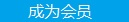您可以捐助，支持我们的公益事业。 1元 10元 50元 认证码：必填求知 文章 文库 Lib 视频 iProcess 课程 角色 咨询 工具 讲座 Modeler Code要资料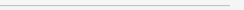订阅捐助
MATLAB从入门到算法实践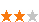802 次浏览     评价： 好 中 差
2018-10-17

 编辑推荐: 本文来自于CSDN，介绍了MATLAB的优缺点、应用场景以及数学建模等相关知识。

MATLAB的优点：

1、 函数多，工具箱全

2、 人性化，且在不断改进

3、 集成度高、文档完善

4、 上手快，表达方式更符合数学模式

Matlab下标从1开始，似伪代码

5、 代码开发时间短

Matlab集成度高

MATLAB的缺点

1、 速度慢

2、 版权

1、 解决实际问题

2、 对数学工具的理解

3、 能力的培养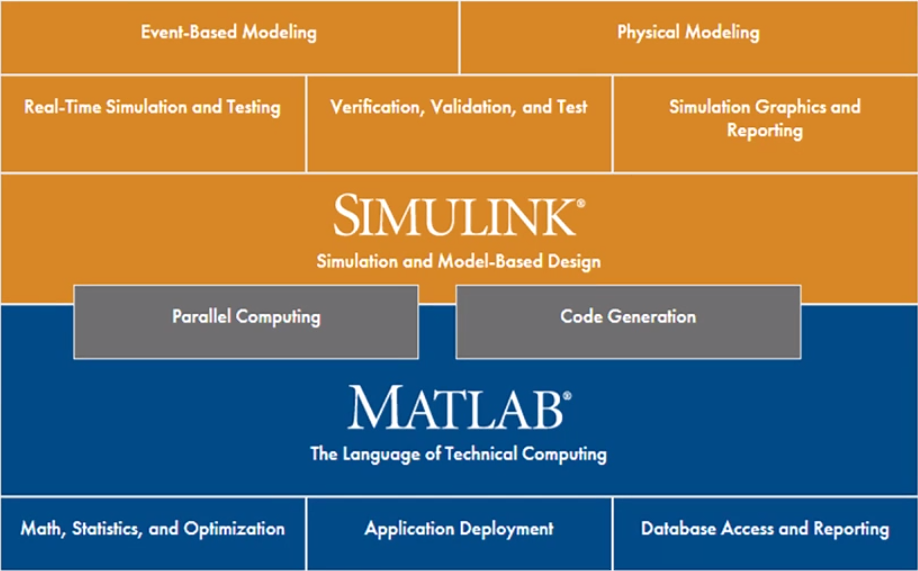- 技术计算。数学计算、分析、可视化和算法开发

- 控制系统设计。控制系统基于模型的设计，包括嵌入式系统

- 仿真、快速原型及代码生成等

- 信号处理和通信。信号处理和通信系统基于模型的设计，包

- 括仿真、代码生成和验证等

- 图像处理。图像采集、分析、可视化和算法开发

- 测试和测量。测试和测量应用中硬件连接性和数据分析

- 计算生物学。生物数据和系统的分析、可视化与仿真

- 计算金融。金融建模、分析、交易及应用程序开发

- 数学建模

1 . 数值计算

 29*(2+23/3)-5^2%数学公式直接得出结果

 a=1%变量赋值运算 b=2 c=a+b

 A = [1 2 3; 4 5 6; 7 8 10] 矩阵，基本运算单元

 rng(0) %设置随机种子，便于读者验证

 A = ones(2, 5) * 6 % 元素全部为6的2*5矩阵

 B = rand(3, 5)

 C = [A; B] % 数组合并

 format long % 长格式显示

 format short % 短格式显示

2 . 矩阵和数组操作

 A=[1 2;3 4]; % 测试矩阵A

 B=[4 3;2 1]; % 测试矩阵B

 r1=100+A % 矩阵A加上一个常数

 r2_1=A*B % 两个矩阵相乘，矩阵乘法

 r2_2=A.*B % 两个矩阵相乘，数组乘法

3 . 稀疏矩阵

 B = bucky;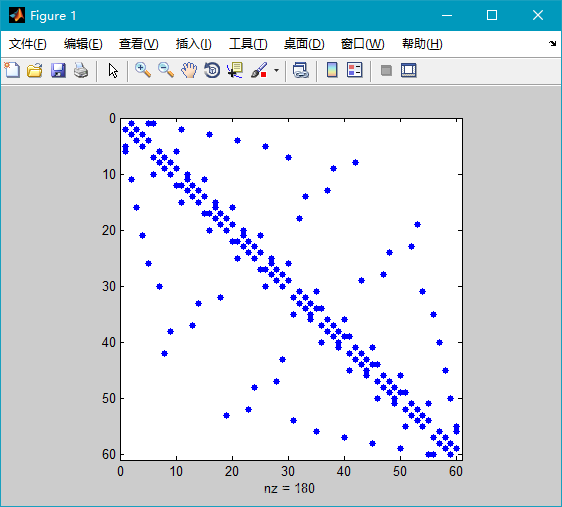spy(B)

 D=full(B)

 A=reshape(1:18,2,3,3) % 三维数组

4.逻辑型

 M=magic(4) sum(M)%每一列的和相等 M>6 %大于6的元素 a='matlab'%字符串

5.结构数组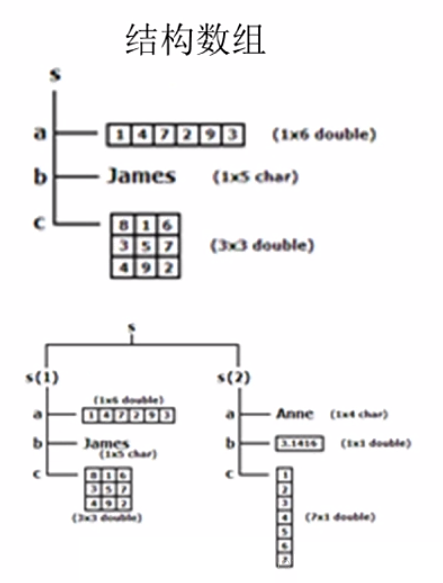employee.name='henry'; employee.sex='male' employee.age=25; employee.number=12345;

 %也可定义二维数组 employee(2).name='lee'; employee(2).sex='female'; employee(2).age=23; employee(2).number=98765; employee(2)

6.元胞数组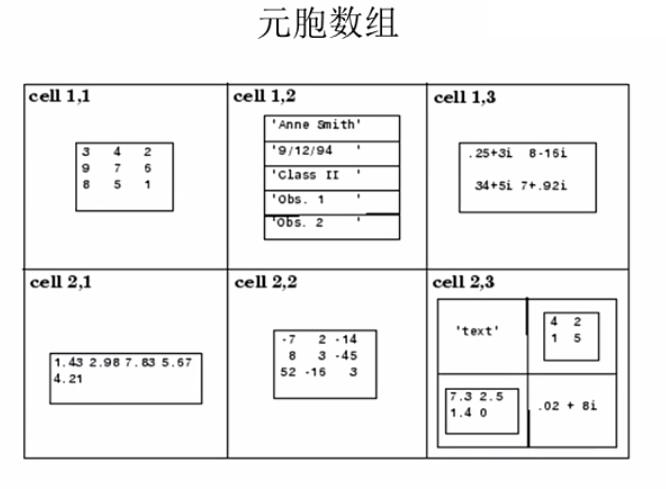7.日期和时间

 t = datetime(2017,6,28,6:7,0,0) datenum(t)%2014b以前的版本适用 datevec(t) t=Day = 27:28%修改日期

matlab可以直接导入excel的数据

table属性，可以通过行列显示也可以通过名称

clc:命令行清除

closeall:绘图窗口关闭

8.差分

 rng(0) %设置随机种子 A=randperm(9) %生成随机数列 B = diff(A) %求数列的差分

9.梯度求解

 v = -2:0.2:2; [x,y] = meshgrid(v); z = x .*exp(-x.^2 - y.^2); %创建测试数据 [px,py] = gradient(z,.2,.2); %求梯度 contour(v,v,z),hold on,quiver(v,v,px,py),hold off %绘制等高线和梯度方向

10.一维差值数组

 x=0:10; y=cos(x); xi=0:0.25:10; strmod={'nearest','linear','spline','pchip'} %将差值方法存储到元胞数组 strlb={'(a)method=nearest','(b)method=linear',... '(c)method=spline','(d)method=pchip'}%绘图标签 for i=1:4 yi=interp1(x,y,xi,strmod{i});%插值 subplot(2,2,i) %子图 plot(x,y,'ro',xi,yi,'b'),xlabel(strlb(i)) end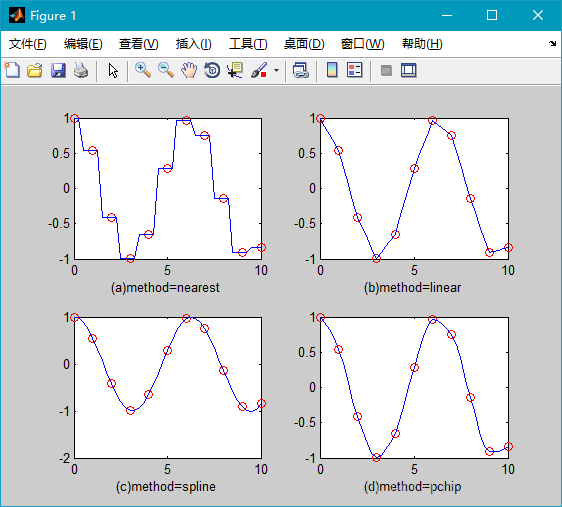11.计算拟合

 rng(0) %设置随机种子 x=1:1:10; y=-0.9*x.^2+10*x+rand(1,10).*5;%产生测试数据 plot(x,y,'o') %绘制并标出原始数据点 p=polyfit(x,y,2) xi=1:0.5:10; yi=polyval(p,xi); %计算拟合的结果 hold on plot(xi,yi); %绘制拟合结果图 hold off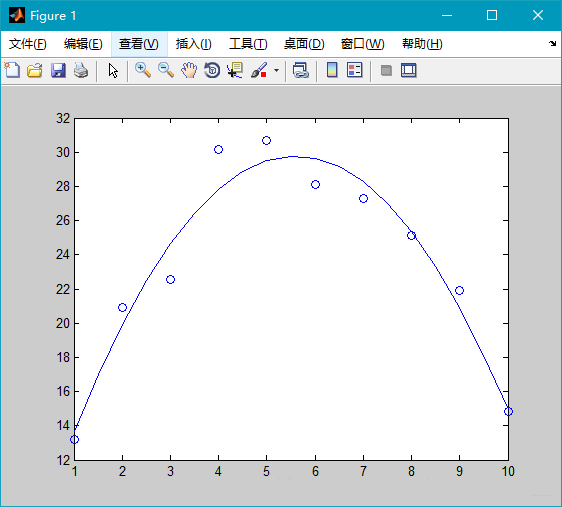12.关于误差

a=0

for n=1:10000

a=a+0.1; % 0.1以二进制存储会有截断误差

end

13.可视化

14.概率分布

15.数据I/O

16.优化算法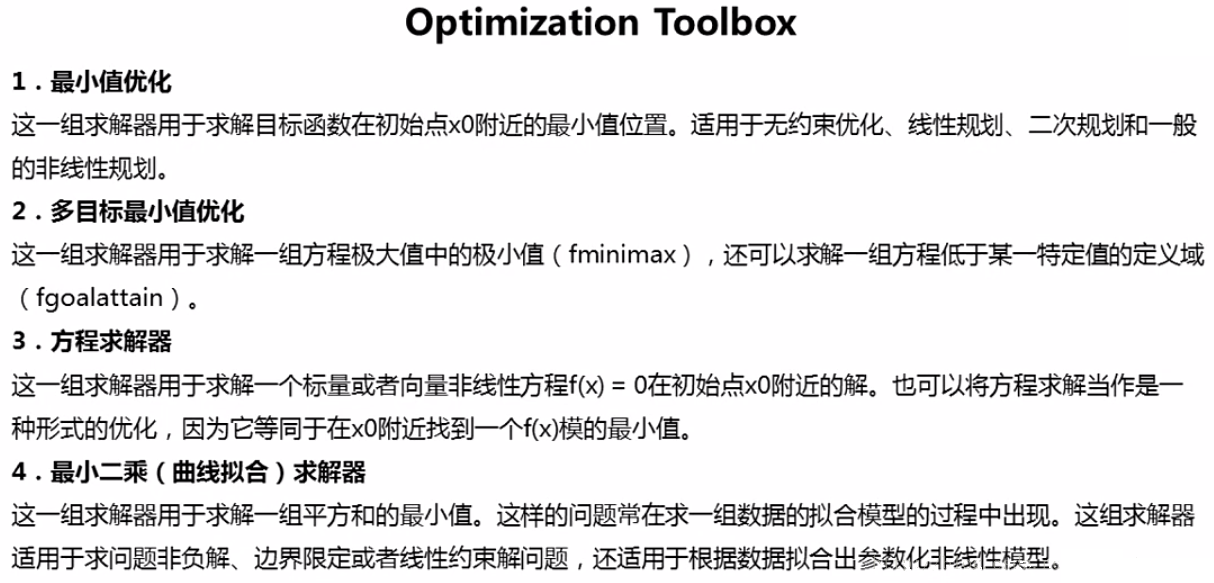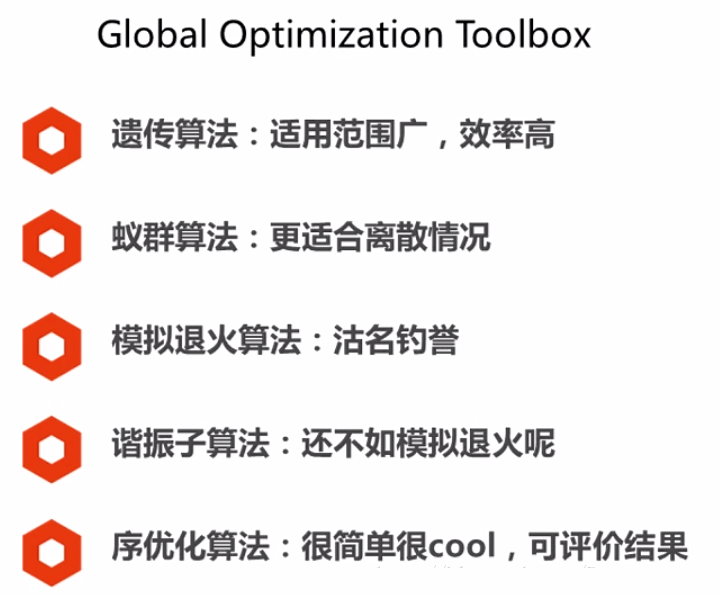17.信号处理

17.1信号处理基本理论

17.1.1信号的生成

17.1.2数字滤波器结构

17.2 IIR滤波器经典设计

17.2.1 IIR滤波器直接设计法

17.2.2 IIR滤波器

17.2.3 广义巴特沃斯IIR滤波器设计

17.3 FIR滤波器的MATLAN实现

17.3.1 FIR滤波器设计

17.3.2 fir1函数

17.3.3 fir2函数

18.1 图像文件的操作

18.1.1 查询图像文件的信息

18.1.2 图像文件的读写

18.1.3 图像文件的显示

18.1.4 图像格式的转换

18.2 图像的几何运算

18.2.1 图像的平移

18.2.2 图像的镜像变换

18.2.3 图像缩放

18.2.4 图像的旋转

18.2.5 图像的剪切

18.3 图像的正交变换

18.3.1 傅里叶变换

18.3.2 离散余弦变换

18.4 MATLAB图像增强

18.4.1 像素值及其统计特性

18.4.2 对比度增强

18.4.3 直方图均衡化

18.4.4 空域滤波增强

18.4.5 频域增强

19.1 机器学习和神经网络

Live Script

Table键可以自动补全

Data Store

MCM99问题：“非法”聚会

MCM03问题：Gamma刀治疗方案

ICM2017问题：优化机场安检口旅客通行（排队问题）

CUMCM14问题：折叠桌

CUMCM16问题：系泊系统的设计

- 请调节重物球的质量，使得钢桶的倾斜角度不超过5度，锚链在锚点与海床的夹角不超过16度

- 水深16-20m，海水速度<1.5m/s，风速<36m/s，请给出考虑风力、水流力和水深情况下的系泊系统设计。802 次浏览  评价: 好 中 差订阅捐助
 相关文章 我们该如何设计数据库 数据库设计经验谈 数据库设计过程 数据库编程总结
 相关文档 数据库性能调优技巧 数据库性能调整 数据库性能优化讲座 数据库系统性能调优系列
 相关课程 高性能数据库设计与优化 高级数据库架构师 数据仓库和数据挖掘技术 Hadoop原理、部署与性能调优每天2个文档/视频 扫描微信二维码订阅
 订阅技术月刊 获得每月300个技术资源